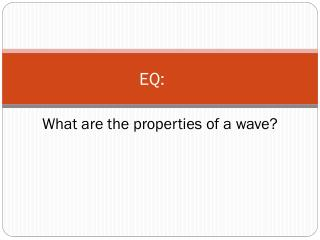DownloadDownload PresentationEQ:

# EQ:

Télécharger la présentation## EQ:

- - - - - - - - - - - - - - - - - - - - - - - - - - - E N D - - - - - - - - - - - - - - - - - - - - - - - - - - -
##### Presentation Transcript

1. EQ: What are the properties of a wave?

2. Wavelength The distance between identical points on an adjacent wave.

3. Crest The highest point on a wave.

4. Amplitude The distance a wave moves from its resting position

5. Trough The lowest point on a wave.

6. Rarefactions Regions in a wave where the particles are farther apart.

7. Compressions Regions where particles bunch together in a wave.

8. Hertz (Hz) Pitch is measured in Hz.  One hertz is equal to one wave per second

9. 4 Basic Properties of a Wave Amplitude Frequency Wavelength Speed

10. Mechanical Waves Require a material medium to travel (air, water, ropes). These waves are divided into three different types.

11. Transverse Waves Transverse waves cause the medium to move perpendicular to the direction of the wave.

12. Longitudinal Waves Longitudinal waves cause the medium to move parallel to the direction of the wave.

13. Surface Waves Surface waves are both transverse waves and longitudinal waves mixed in one medium.

14. Frequency The number of complete waves that pass a given point in a certain amount of time.